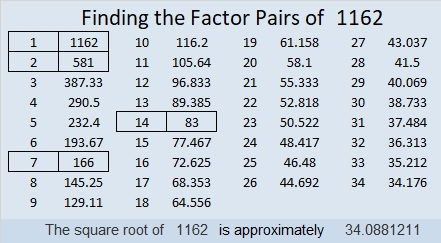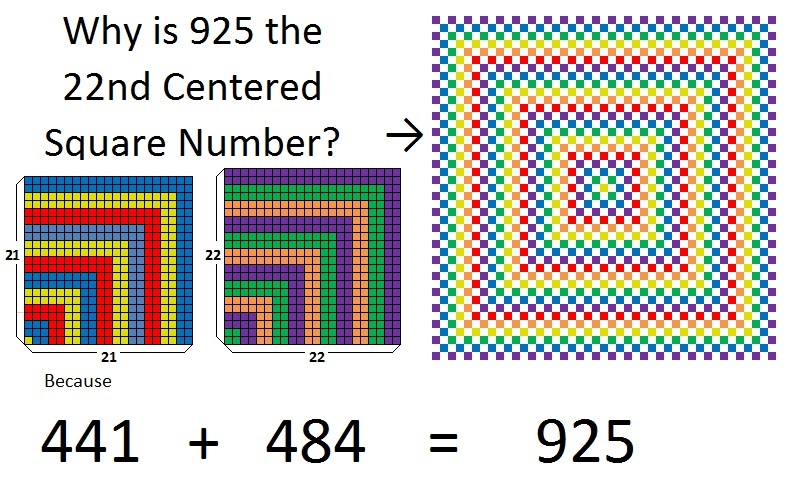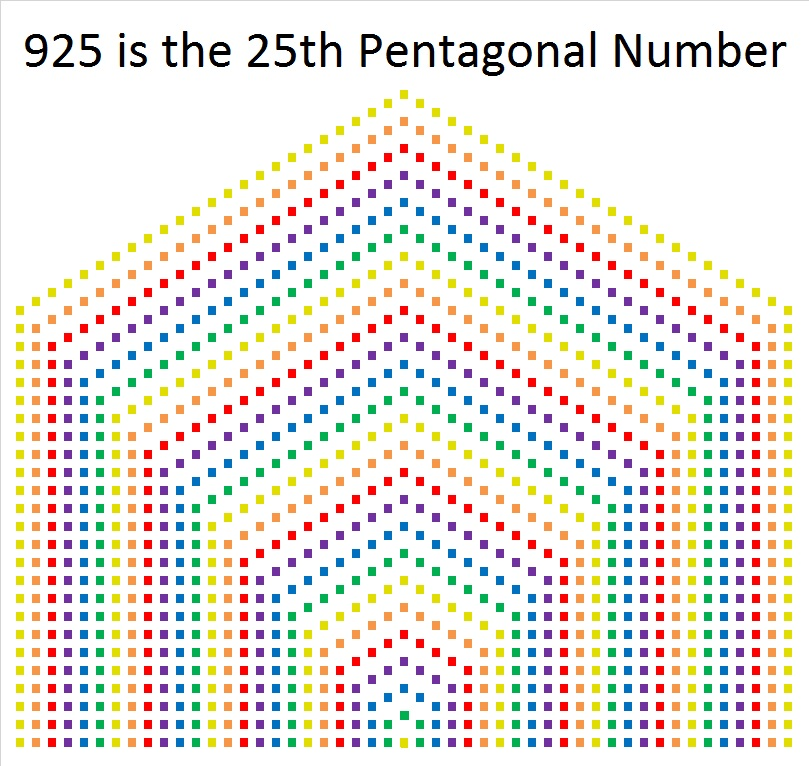# 1162 Is a Pentagonal Number

1162 is the 28th pentagonal number because (3(28²) – 28)/2 = 1162. I created the graphic below so you can see all the pentagonal numbers up to 1162 and figure out why all of them are called pentagonal numbers.Here’s more about the number 1162:

• 1162 is a composite number.
• Prime factorization: 1162 = 2 × 7 × 83
• The exponents in the prime factorization are 1, 1, and 1. Adding one to each and multiplying we get (1 + 1)(1 + 1)(1 + 1) = 2 × 2 × 2 = 8. Therefore 1162 has exactly 8 factors.
• Factors of 1162: 1, 2, 7, 14, 83, 166, 581, 1162
• Factor pairs: 1162 = 1 × 1162, 2 × 581, 7 × 166, or 14 × 83
• 1162 has no square factors that allow its square root to be simplified. √1162 ≈ 34.088121162 is a palindrome in three bases:
It’s 343 in BASE 19 because 3(19²) + 4(19) + 3(1) = 1162,
2I2 in BASE 20 (I is 18 base 10) because 2(20²) + 18(20) + 2(1) = 1162, and
1G1 in BASE 27 (G is 16 base 10) because 27² + 16(27) + 1 = 1162

# What Kind of Shape is 925 In?

925 is the 22nd Centered Square number because 22² + 21² = 925. I made this graphic to show this fact through the use of color. Look at the center of the centered square. Can you see how the single yellow square and the four small green squares in the center correspond to the same colored squares in the smaller squares? The pattern continues from the inside of the centered square to the outside.925 is the sum of two squares these THREE ways:

• 22² + 21² = 925
• 27² + 14² = 925
• 30² + 5² = 925

925 is the hypotenuse of SEVEN Pythagorean triples:

• 43-924-925
• 259-888-925
• 285-880-925
• 300-875-925
• 520-765-925
• 533-756-925
• 555-740-925

925 is the 25th pentagonal number because 3((25²) – 25)/2 = 925. The shape in the graphic below of 925 tiny squares may look more like a house, but it is still very much a pentagon.925 looks interesting in a few other bases:

4141 in BASE 6
1K1 BASE 22 (K is 20 in base 10)
151 BASE 28
PP in BASE 36 (P is 25 in base 10)

• 925 is a composite number.
• Prime factorization: 925 = 5 × 5 × 37, which can be written 925 = 2² × 37
• The exponents in the prime factorization are 2 and 1. Adding one to each and multiplying we get (2 + 1)(1 + 1) = 3 × 2  = 6. Therefore 925 has exactly 6 factors.
• Factors of 925: 1, 5, 25, 37, 185, 925
• Factor pairs: 925 = 1 × 925, 5 × 185, or 25 × 37
• Taking the factor pair with the largest square number factor, we get √925 = (√25)(√37) = 5√37 ≈ 30.41381